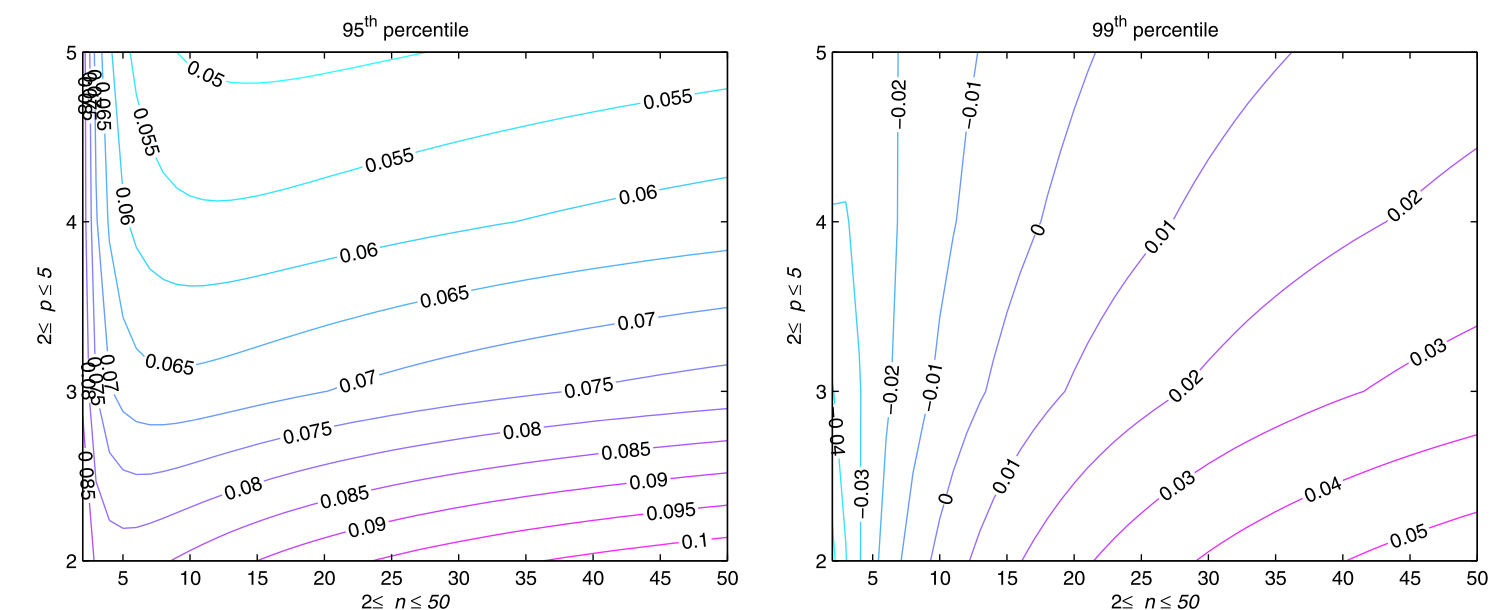# Rates of convergence to Tracy-Widom?

$$\renewcommand{\!}{\mathbf} \renewcommand{\Ai}{\operatorname{Ai}}$$ One can define the Tracy-Widom distribution as the Fredholm determinant $$F_2(t)=\det(\mathbf I-\mathbf A)$$ where $$\mathbf A(x, y)=\begin{cases} \frac{\Ai(x) \Ai'(y)-\Ai'(x) \Ai(y)}{x-y} & \text {if } x \neq y \\ \Ai'(x)^{2}-x \Ai(x)^{2} & \text {if } x=y \end{cases} \text{ for } \Ai(x) = \frac 1{\pi} \int_0^\infty \cos\Big(\tfrac 13 t^3 + xt\Big) \, d t$$ It's well known that the largest eigenvalue of an $$n\times n$$ GUE matrix (appropriately scaled) converges in distribution to $$F_2$$. Also, by the celebrated Baik-Deft-Johansson theorem, the length of the longest increasing subsequence of a random permutation $$\in S_n$$ (appropriately scaled) also converges in distribution to $$F_2$$. I'm sure there are many other examples of situations in which $$F_2$$ appears as the limiting distribution.

However, I have been trying to find references about how fast the things that converge to $$F_2$$ converge to $$F_2$$. My searches have turned up this paper: https://arxiv.org/pdf/0803.3408.pdf and this paper: https://arxiv.org/pdf/1901.05235.pdf, both of which seem to answer related questions but not exactly what I'm looking for.

Does anyone know the rates at which the largest eigenvalue of a GUE matrix and/or the longest increasing subsequence of a random permutation converge to $$F_2$$? If not exactly, are there references that have done numerical computations and put forward a conjecture about such rates?

• I think it is an open question to design a Stein's method framework when the limiting distribution is a Tracy-Widom one. – user69642 May 26 '20 at 7:35

Accuracy of the Tracy-Widom limits for the extreme eigenvalues in white Wishart matrices studies the rate of convergence for Wishart matrices $$WW^T$$, with $$W$$ an $$n\times p$$ matrix with i.i.d. Gaussian matrix elements. The error is $${\cal O}[\min(n,p)]^{-2/3}$$.